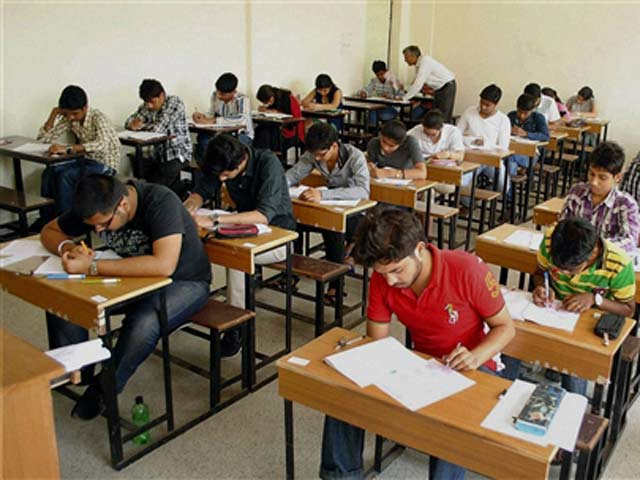May 17, 2021

# Top Universities Across India Give Incorrect Equivalent Percentage Of CGPA To Students, Need For Universal FormulaDr HR Bhapkar

Pune, January 30, 2021: University Grants Commission (UGC) should bring guidelines to have one parameter for converting percentage to CGPA. Presently, most of the institutions or academia are using the choice based credit system to grade the students according to their intelligence.

Before that, a student would be judged through the mark s/he has obtained in the examination. The marking system would help the government offices and industries to categorise the students according to their merit.

Although the CGPA system is considered to be the better system to evaluate a student, the lacuna lies with the conversion of CGPA to a percentage as almost all the universities and institutions have different methods of this conversion.

This disparity needs to be removed for better implementation of  Choice Based Credit System (CBCS), particularly in terms of deciding the merit of the student.

After studying it I found that there are at least nine different formulae to convert CGPA to percentage in Indian Universities. The same CGPA is converted to different percentages as per formulae used by the university. For example, 9.0 CGPA of a student is converted to percentages from 76.25 % to 95 % as per the rules framed by the universities. The following table depicts the disparity in the conversion of CGPA to percentages.

The following table depicts the disparity in conversion of CGPA to percentages:

 A B C D E F G H I J K Sr. No. University/ Institute % of CGPA = 10 % of CGPA = 9.5 % of CGPA = 9 % of CGPA = 8.5 % of CGPA = 8 % of CGPA = 7 % of CGPA = 6 % of CGPA = 5 % of CGPA = 4 1 IIT, Kharagpur 100 95 90 85 80 70 60 50 40 2 Indian Institute of Space Science and Technology 95 90 85 80 75 65 55 45 35 3 Indian Institute of India, Rooraki 95 95 95 90 85 75 65 55 45 4 University of Delhi 95 90.25 85.5 80.75 76 66.5 57 47.5 38 5 Visvesvaraya Technologocal University, Karnataka 92.5 87.5 82.5 77.5 72.5 62.5 52.5 42.5 32.5 6 Dr. Babasaheb Ambedkar Technological University, Lonere 92.31 87.69 83.08 78.46 73.85 64.62 55.38 46.15 36.92 7 Maharashtra National Law University, Nagpur 90 85.5 81 76.5 72 63 54 45 36 8 Savitribai Phule Pune University, Pune 88 83.6 79.2 74.8 70.4 61.6 52.8 44 35.2 9 University of Mumbai 83.5 79.88 76.25 72.63 69 61.75 54.5 47.25 40

If a student secures more than 95 marks in all subjects, then his/her CGPA will be 10 and the percentage must be more than 95. So how can the universities give him/her 83.5 % or 88 %? As the universities/institutes/colleges are using a different formula for conversion of CGPA to the percentage, there is a disparity in terms of percentage. In fact, for the same CGPA, there has to be the same ‘Percentage’ across the country.

I, therefore, recommend that UGC should consider the formula suggested by me to remove the disparity. UGC may bring out the guidelines which must be followed by the universities/ institutes/ colleges across India. Thus it will help employers or academia to compare the merit easily on the same platform. At the same time, it will remove the injustice being done to the students.

I have suggested two formulae:
(1) Formula 1: Actual Percentage: ‘Weighted percentage should be used’ instead of a simple percentage. However, it is difficult to use it from the implementation point of view.
(2) Formula 2: CGPA to Percentage Conversion Formula: Percentage = CGPA * 10 for 0-4 point scale credit system.

The ‘Formula 2’ is easy from a calculation point of view similarly there won’t be any difficulty in implementing it at the practical level. Hence, I strongly recommend that UGC should consider this formula while preparing the guidelines on ‘Conversion of CGPA to Percentage’ and uniform ingredients of the credit system.

This will remove the ‘disparity’ in determining the equivalence of ‘CGPA’ to ‘Percentage’. Hope my research findings will help all the educational institutes across India in removing the disparity and give the same justice to all the student stakeholders.

(Dr H R Bhapkar is an Associate Professor at Department of Mathematics, MIT ADT University’s MIT School of Engineering, Loni Kalbhor, Pune.)